# Two-way ANOVA in SPSS Statistics

## Introduction

The two-way ANOVA compares the mean differences between groups that have been split on two independent variables (called factors). The primary purpose of a two-way ANOVA is to understand if there is an interaction between the two independent variables on the dependent variable. For example, you could use a two-way ANOVA to understand whether there is an interaction between gender and educational level on test anxiety amongst university students, where gender (males/females) and education level (undergraduate/postgraduate) are your independent variables, and test anxiety is your dependent variable. Alternately, you may want to determine whether there is an interaction between physical activity level and gender on blood cholesterol concentration in children, where physical activity (low/moderate/high) and gender (male/female) are your independent variables, and cholesterol concentration is your dependent variable.

The interaction term in a two-way ANOVA informs you whether the effect of one of your independent variables on the dependent variable is the same for all values of your other independent variable (and vice versa). For example, is the effect of gender (male/female) on test anxiety influenced by educational level (undergraduate/postgraduate)? Additionally, if a statistically significant interaction is found, you need to determine whether there are any "simple main effects", and if there are, what these effects are (we discuss this later in our guide).

Note: If you have three independent variables rather than two, you need a three-way ANOVA. Alternatively, if you have a continuous covariate, you need a two-way ANCOVA.

In this "quick start" guide, we show you how to carry out a two-way ANOVA using SPSS Statistics, as well as interpret and report the results from this test. However, before we introduce you to this procedure, you need to understand the different assumptions that your data must meet in order for a two-way ANOVA to give you a valid result. We discuss these assumptions next.

## Assumptions

When you choose to analyse your data using a two-way ANOVA, part of the process involves checking to make sure that the data you want to analyse can actually be analysed using a two-way ANOVA. You need to do this because it is only appropriate to use a two-way ANOVA if your data "passes" six assumptions that are required for a two-way ANOVA to give you a valid result. In practice, checking for these six assumptions means that you have a few more procedures to run through in SPSS Statistics when performing your analysis, as well as spend a little bit more time thinking about your data, but it is not a difficult task.

Before we introduce you to these six assumptions, do not be surprised if, when analysing your own data using SPSS Statistics, one or more of these assumptions is violated (i.e., is not met). This is not uncommon when working with real-world data rather than textbook examples, which often only show you how to carry out a two-way ANOVA when everything goes well! However, don’t worry. Even when your data fails certain assumptions, there is often a solution to overcome this. First, let’s take a look at these six assumptions:

• Assumption #1: Your dependent variable should be measured at the continuous level (i.e., they are interval or ratio variables). Examples of continuous variables include revision time (measured in hours), intelligence (measured using IQ score), exam performance (measured from 0 to 100), weight (measured in kg), and so forth. You can learn more about interval and ratio variables in our article: Types of Variable.
• Assumption #2: Your two independent variables should each consist of two or more categorical, independent groups. Example independent variables that meet this criterion include gender (2 groups: male or female), ethnicity (3 groups: Caucasian, African American and Hispanic), profession (5 groups: surgeon, doctor, nurse, dentist, therapist), and so forth.
• Assumption #3: You should have independence of observations, which means that there is no relationship between the observations in each group or between the groups themselves. For example, there must be different participants in each group with no participant being in more than one group. This is more of a study design issue than something you would test for, but it is an important assumption of the two-way ANOVA. If your study fails this assumption, you will need to use another statistical test instead of the two-way ANOVA (e.g., a repeated measures design). If you are unsure whether your study meets this assumption, you can use our Statistical Test Selector, which is part of our enhanced guides.
• Assumption #4: There should be no significant outliers. Outliers are data points within your data that do not follow the usual pattern (e.g., in a study of 100 students' IQ scores, where the mean score was 108 with only a small variation between students, one student had a score of 156, which is very unusual, and may even put her in the top 1% of IQ scores globally). The problem with outliers is that they can have a negative effect on the two-way ANOVA, reducing the accuracy of your results. Fortunately, when using SPSS Statistics to run a two-way ANOVA on your data, you can easily detect possible outliers. In our enhanced two-way ANOVA guide, we: (a) show you how to detect outliers using SPSS Statistics; and (b) discuss some of the options you have in order to deal with outliers.
• Assumption #5: Your dependent variable should be approximately normally distributed for each combination of the groups of the two independent variables. Whilst this sounds a little tricky, it is easily tested for using SPSS Statistics. Also, when we talk about the two-way ANOVA only requiring approximately normal data, this is because it is quite "robust" to violations of normality, meaning the assumption can be a little violated and still provide valid results. You can test for normality using the Shapiro-Wilk test for normality, which is easily tested for using SPSS Statistics. In addition to showing you how to do this in our enhanced two-way ANOVA guide, we also explain what you can do if your data fails this assumption (i.e., if it fails it more than a little bit).
• Assumption #6: There needs to be homogeneity of variances for each combination of the groups of the two independent variables. Again, whilst this sounds a little tricky, you can easily test this assumption in SPSS Statistics using Levene’s test for homogeneity of variances. In our enhanced two-way ANOVA guide, we (a) show you how to perform Levene’s test for homogeneity of variances in SPSS Statistics, (b) explain some of the things you will need to consider when interpreting your data, and (c) present possible ways to continue with your analysis if your data fails to meet this assumption.

You can check assumptions #4, #5 and #6 using SPSS Statistics. Before doing this, you should make sure that your data meets assumptions #1, #2 and #3, although you don’t need SPSS Statistics to do this. Just remember that if you do not run the statistical tests on these assumptions correctly, the results you get when running a two-way ANOVA might not be valid. This is why we dedicate a number of sections of our enhanced two-way ANOVA guide to help you get this right. You can find out about our enhanced content as a whole here, or more specifically, learn how we help with testing assumptions here.

In the section, Test Procedure in SPSS Statistics, we illustrate the SPSS Statistics procedure to perform a two-way ANOVA assuming that no assumptions have been violated. First, we set out the example we use to explain the two-way ANOVA procedure in SPSS Statistics.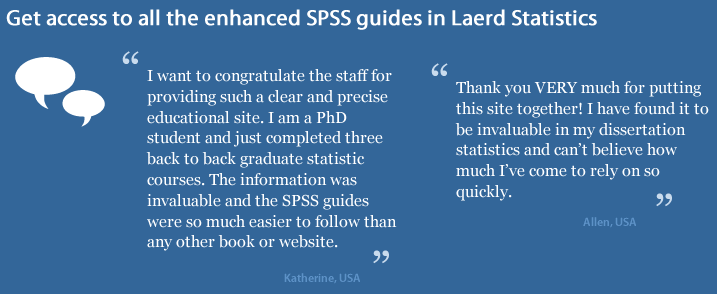## Example

A researcher was interested in whether an individual's interest in politics was influenced by their level of education and gender. They recruited a random sample of participants to their study and asked them about their interest in politics, which they scored from 0 to 100, with higher scores indicating a greater interest in politics. The researcher then divided the participants by gender (Male/Female) and then again by level of education (School/College/University). Therefore, the dependent variable was "interest in politics", and the two independent variables were "gender" and "education".

## Setup in SPSS Statistics

In SPSS Statistics, we separated the individuals into their appropriate groups by using two columns representing the two independent variables, and labelled them Gender and Edu_Level. For Gender, we coded "males" as 1 and "females" as 2, and for Edu_Level, we coded "school" as 1, "college" as 2 and "university" as 3. The participants' interest in politics – the dependent variable – was entered under the variable name, Int_Politics. The setup for this example can be seen below: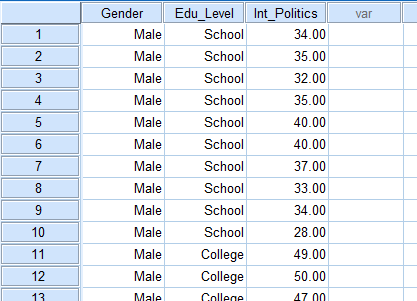Published with written permission from SPSS Statistics, IBM Corporation.

If you are still unsure how to correctly set up your data in SPSS Statistics to carry out a two-way ANOVA, we show you all the required steps in our enhanced two-way ANOVA guide.

## Test Procedure in SPSS Statistics

The 14 steps below show you how to analyse your data using a two-way ANOVA in SPSS Statistics when the six assumptions in the previous section, Assumptions, have not been violated. At the end of these 14 steps, we show you how to interpret the results from this test. If you are looking for help to make sure your data meets assumptions #4, #5 and #6, which are required when using a two-way ANOVA and can be tested using SPSS Statistics, you can learn more in our enhanced guides here.

• Click Analyze > General Linear Model > Univariate... on the top menu, as shown below: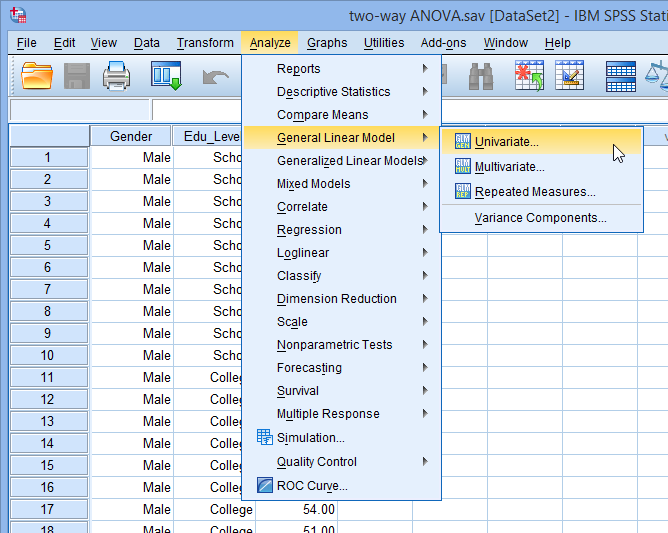Published with written permission from SPSS Statistics, IBM Corporation.

• You will be presented with the Univariate dialogue box, as shown below: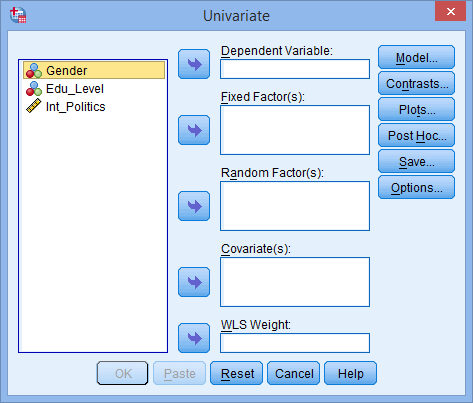Published with written permission from SPSS Statistics, IBM Corporation.

• Transfer the dependent variable, Int_Politics, into the Dependent Variable: box, and transfer both independent variables, Gender and Edu_Level, into the Fixed Factor(s): box. You can do this by drag-and-dropping the variables into the respective boxes or by using the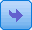button. If you are using older versions of SPSS Statistics you will need to use the latter method. You will end up with a screen similar to that shown below: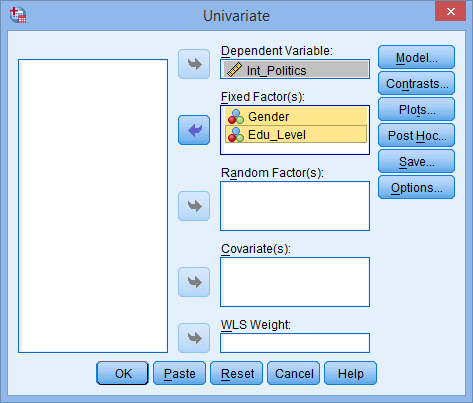Published with written permission from SPSS Statistics, IBM Corporation.

Note: For this analysis, you will not need to worry about the Random Factor(s):, Covariate(s): or WLS Weight: boxes.

• Click on the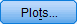button. You will be presented with the Univariate: Profile Plots dialogue box, as shown below: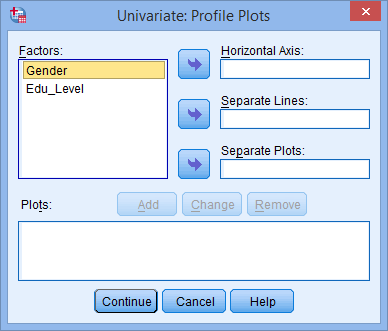Published with written permission from SPSS Statistics, IBM Corporation.

• Transfer the independent variable, Edu_Level, from the Factors: box into the Horizontal Axis: box, and transfer the other independent variable, Gender, into the Separate Lines: box. You will be presented with the following screen: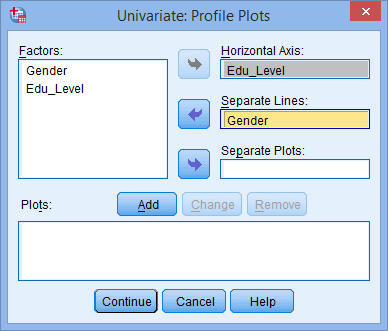Published with written permission from SPSS Statistics, IBM Corporation.

Note: It can help to put the independent variable with the greater number of groups in the Horizontal Axis: box.

• Click the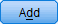button. You will see that "Edu_Level*Gender" has been added to the Plots: box, as shown below: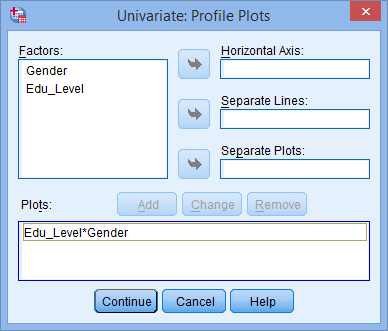Published with written permission from SPSS Statistics, IBM Corporation.

Join the 10,000s of students, academics and professionals who rely on Laerd Statistics.
• Click the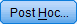button. You will be presented with the Univariate: Post Hoc Multiple Comparisons for Observed Means dialogue box, as shown below: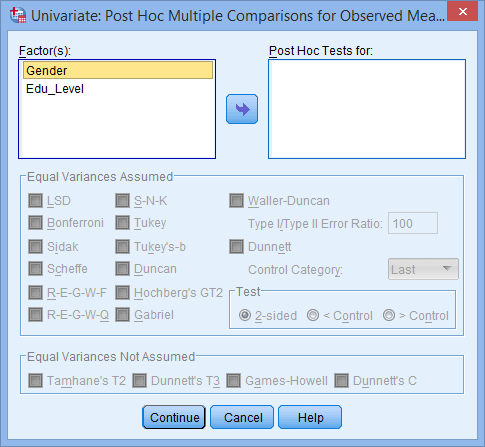Published with written permission from SPSS Statistics, IBM Corporation.

• Transfer Edu_Level from the Factor(s): box to the Post Hoc Tests for: box. This will make the –Equal Variances Assumed– area become active (lose the "grey sheen") and present you with some choices for which post hoc test to use. For this example, we are going to select Tukey, which is a good, all-round post hoc test.

Note: You only need to transfer independent variables that have more than two groups into the Post Hoc Tests for: box. This is why we do not transfer Gender.

You will finish up with the following screen: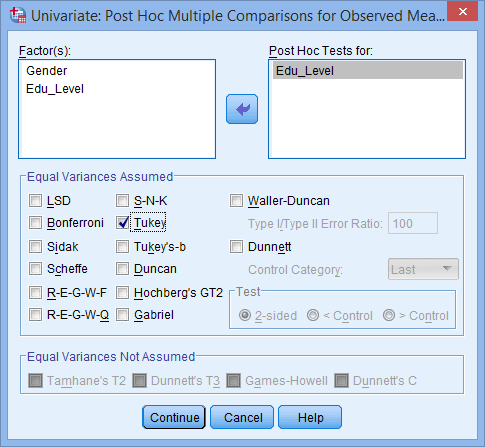Published with written permission from SPSS Statistics, IBM Corporation.

• Click the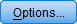button. This will present you with the Univariate: Options dialogue box, as shown below: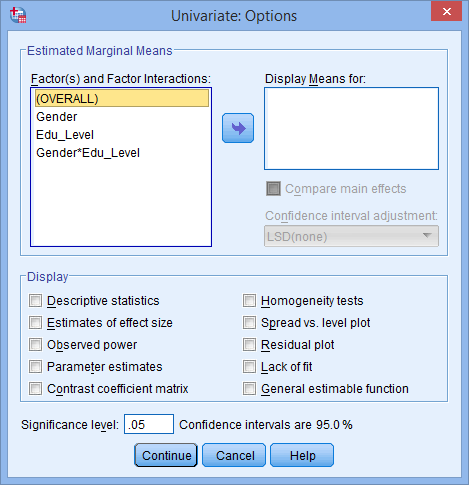Published with written permission from SPSS Statistics, IBM Corporation.

• Transfer Gender, Edu_Level and Gender*Edu_Level from the Factor(s) and Factor Interactions: box into the Display Means for: box. In the –Display– area, tick the Descriptive Statistics option. You will presented with the following screen: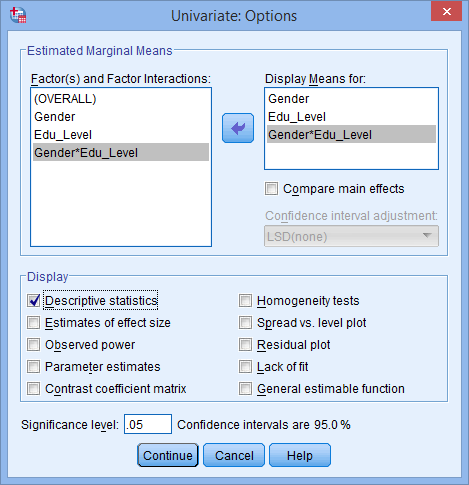Published with written permission from SPSS Statistics, IBM Corporation.

Go to the next page for the SPSS Statistics output, discussion of simple main effects and an explanation of the output.